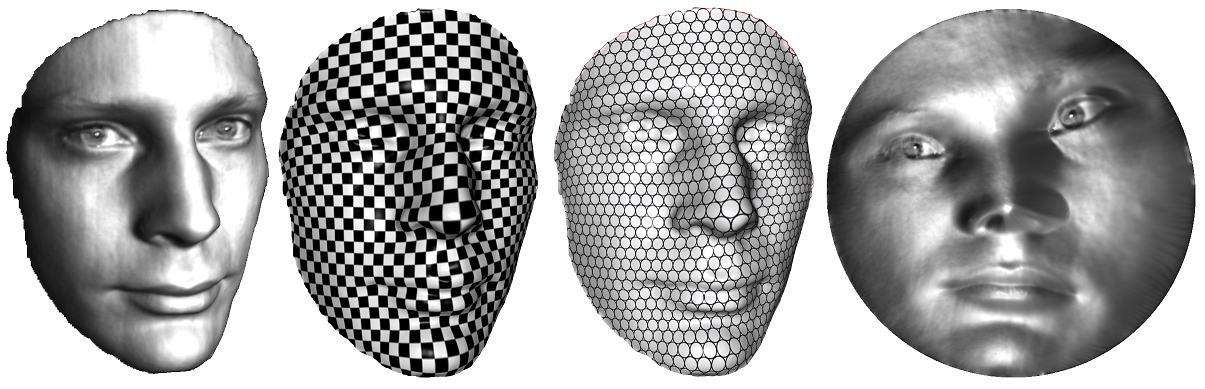# Riemann Mapping Tutorial with C++ Source

### Conformal Mapping

Suppose $S$ is an orientable surface embedded in the Euclidea space $R^3$. The surface has an induced Euclidean metric $\mathbf{g}$. Let $D$ be another metric surface with a Riemannian metric $\mathbf{h}$. Let $\phi: (S,\mathbf{g})\to (D,\mathbf{h})$ be a mapping. Let $\gamma:[0,1]\to S$ be a curve on the surface $S$, its image $\phi(\gamma)$ is a curve on the target surface $D$. We can define the length of $\gamma$ on $S$ by the length of $\phi(\gamma)$ on $D$, this gives the concept of pull back metric. Suppose $(x,y)$ is the local coordinates of $S$, $(u,v)$ is the local coordinates of $D$, the mapping $\phi$ can be represented as $\phi:(x,y)\to (u(x,y),v(x,y)).$ Then the Jacobin matrix of the mapping is given by $\left(\begin{array}{c} du \\dv \end{array}\right) = \left(\begin{array}{cc} u_x & u_y\\ v_x & v_y \end{array}\right)\left(\begin{array}{c} dx \\dy \end{array}\right)$ The metric $\mathbf{h}$ has local representation as $\mathbf{h}(u,v) = \left(\begin{array}{cc} du &dv \end{array}\right) \left(\begin{array}{cc} h_{11}& h_{12}\\h_{21}&h_{22} \end{array}\right) \left(\begin{array}{c} du \\dv \end{array}\right)$ The pull back metric induced by $\phi$ has local representation as $\phi^*\mathbf{h} = \left(\begin{array}{cc} dx &dy \end{array}\right) \left(\begin{array}{cc} u_x & u_y\\ v_x & v_y \end{array}\right)^T \left(\begin{array}{cc} h_{11}& h_{12}\\h_{21}&h_{22} \end{array}\right) \left(\begin{array}{cc} u_x & u_y\\ v_x & v_y \end{array}\right) \left(\begin{array}{c} dx \\dy \end{array}\right)$ We say the mapping $\phi$ is conformal (angle preserving), if the pull back metric and the original metric differ by a scalar function $\phi^*\mathbf{h} = e^{2\lambda} \mathbf{g},$ where the function $\lambda:S\to R$ is called conformal factor function, which gives the area distortion factor. A conformal mapping preserves the angles, and maps infinitesimal circles to the infinitesimal circles, as shown in the top figure.

### Riemann Mapping

Riemann Mapping Theorem If $S$ is a topological disk, and $D$ is the planar disk, then there exist conformal mappings from the surface to the disk. All such mappings differ by a Mobius transformation.

A Mobius transformation conformally maps the unit disk to itself, and has the closed form $\phi(z) = e^{i\theta} \frac{z-z_0}{1-\bar{z}_0 z}, |z| < 1.$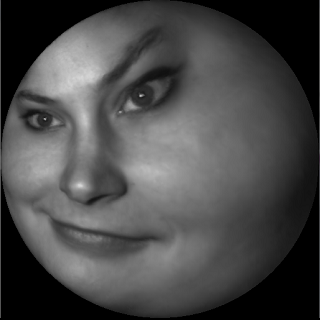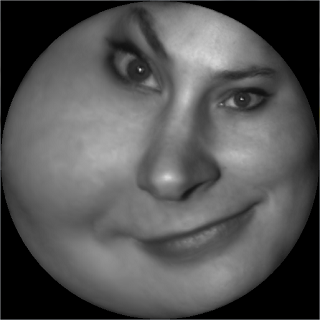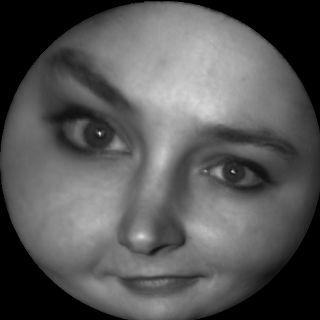## Simplicial Forms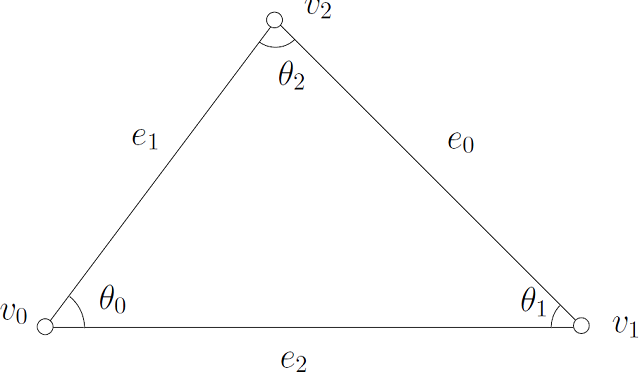Suppose $M$ is a simplicial complex. The simplicial 0-form is a function defined on the vertices, the simplicial 1-form is a linear function defined on the halfedges, the simplicial 2-form is a linear function defined on the oriented faces.

### Exterior Differentiation

Suppose $f: V\to R$ is a 0-form, then $df$ is a 1-form, $df( [v_0,v_1]) = f(\partial [v_0,v_1]) = f(v_1) - f(v_0).$ Suppose $\omega$ is a 1-form, then $d\omega$ is a 2-form $d\omega([v_0,v_1,v_2]) = \omega (\partial [v_0,v_1,v_2]) = \omega([v_0,v_1]) + \omega([v_1,v_2]) + \omega ([v_2,v_0]).$

### Wedge Product

Let $\omega_1$ and $\omega_2$ are two closed 1-forms, the wedge product $\omega_1\wedge \omega_2$ integrated on the triangle $[v_0,v_1,v_2]$ equals to $\int_{[v_0,v_1,v_2]} \omega_1 \wedge \omega_2 = \frac{1}{6} \left| \begin{array}{ccc} \omega_1(e_0) &\omega_1(e_1) &\omega_1(e_2) \\ \omega_2(e_0) &\omega_2(e_1) &\omega_2(e_2) \\ 1 & 1 & 1 \end{array} \right|$

### Wedge Star Product

The integration of the star wedge product $\omega_1 \wedge {}^*\omega_2$ on $[v_0,v_1,v_2]$ equals to $\int_{[v_0,v_1,v_2]} \omega_1 \wedge {}^*\omega_2 = \frac{1}{2} \sum_{k=0}^2 \cot(\theta_k) \omega_1(e_k) \omega_2(e_k).$

### Harmonic 1-form

Suppose $\omega$ is a 1-form, $\omega$ is harmonic, if it satisfies $d\omega = 0, \delta \omega = 0,$ where $\delta \omega(v_i) = \sum_{[v_i,v_j]\in M} w_{ij} \omega([v_i,v_j]), \forall v_i\in M,$ where $w_{ij}$ is the cotangent edge weight.

### Diffusion

According to Hodge theory, every cohomological class has a unique harmonic 1-form. Suppose $\omega$ is a closed 1-form, then there exists a unique function (0-form) $f: V\to R$, such that $\omega + df$ is harmonic. Namely, $d(\omega+df) = d\omega + d^2f = 0, \delta (\omega+ df ) = 0,$ The second equation is equivalent to $\sum_{j} w_{ij}(f(v_j) - f(v_i)) = -\sum_j w_{ij}\omega([v_i,v_j]), \forall v_i.$

### Computational Algorithm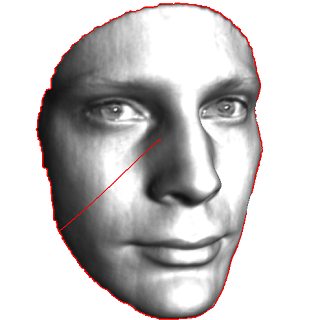The input mesh $M$ is a topological disk, like a human face mesh.
1. Punch a hole in the center of the surface. The surface becomes an annulus, with two boundaries $\partial M = \gamma_0 - \gamma_1.$
2. Compute the shortest path $\eta$ connecting $\gamma_0$ and $\gamma_1$. Slice the surface along $\eta$ to get a topological disk $\bar{M}$, $\partial \bar{M} = \gamma_0 \cup \eta^{+} \cup \gamma_1 \cup \eta^{-1}.$
3.Exact harmonic 1-form.
4. Compute the exact harmonoic 1-form $\omega_0$. Compute a harmonic function by solving the following Dirichlet problem, $\left\{ \begin{array}{clc} \Delta f &=& 0\\ f|_{\gamma_0} & =& 0\\ f|_{\gamma_1} & =& 1 \end{array} \right.$ on the mesh, the discrete harmonic function can be computed $\left\{ \begin{array}{rlcr} \sum_{j} w_{ij}(f(v_i) - f(v_j)) &=& 0 & \forall v_i \in M\\ f(v_k) & =& 0 & \forall v_k \in \gamma_0\\ f(v_l) & =& 1 & \forall v_l \in \gamma_1\\ \end{array} \right.$ Then the exact harmonic 1-form is given by $\omega_0 = df.$
5. Assign a function on the sliced mesh $g: \bar{M}\to R$, such that $g(v_i) = \left\{ \begin{array}{cl} 1 & \forall v_i \in \eta^{+}\\ 0 & otherwise \end{array} \right.$ then suppose $e^{+}\in \eta^{+}$, its dual $e^{-}\in \eta^{-}$. Because $dg(e^{+}) = dg(e^{-})=0$, so $dg$ is well defined on the annulus $M$. Define $\tau = dg, \int_{\gamma_0} \tau = 1.$
6.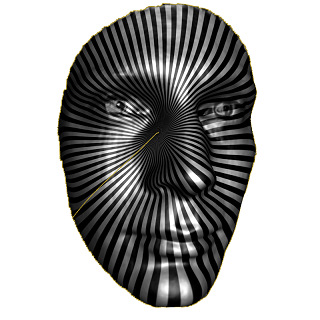Closed harmonic 1-form.
7. Diffusion Compute a function $h:V\to R$, such that $\tau + dh$ is harmonic. $\sum_{j} w_{ij} [ \tau( [v_i,v_j] ) + h(v_j) - h(v_i) ] = 0$ set $\omega_1 = \tau + dh$.
8.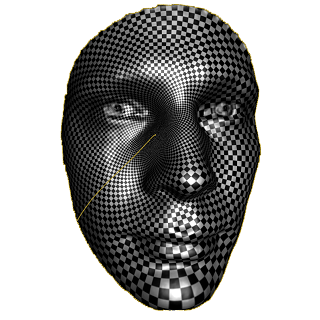Holomorphic 1-form.
9. Holomorphic 1-form Now we have two harmonic 1-forms $\omega_0$ and $\omega_1$. $\left( \begin{array}{c} {}^*\omega_0 \\ {}^*\omega_1 \end{array} \right) = \left( \begin{array}{cc} \lambda_{00} & \lambda_{01}\\ \lambda_{10} & \lambda_{11} \end{array} \right) \left( \begin{array}{c} \omega_0 \\ \omega_1 \end{array} \right)$ We obtain linear system $\left( \begin{array}{c} \int_M \omega_0 \wedge {}^*\omega_0 & \int_M \omega_0 \wedge {}^*\omega_1 \\ \int_M \omega_1 \wedge {}^*\omega_0 & \int_M \omega_1 \wedge {}^*\omega_1 \end{array} \right) = \left( \begin{array}{cc} \int_M \omega_0 \wedge \omega_0 & \int_M \omega_0 \wedge \omega_1 \\ \int_M \omega_1 \wedge \omega_0 & \int_M \omega_1 \wedge \omega_1 \\ \end{array} \right) \left( \begin{array}{c} \lambda_{00} & \lambda_{10}\\ \lambda_{01} & \lambda_{11} \end{array} \right)$
10.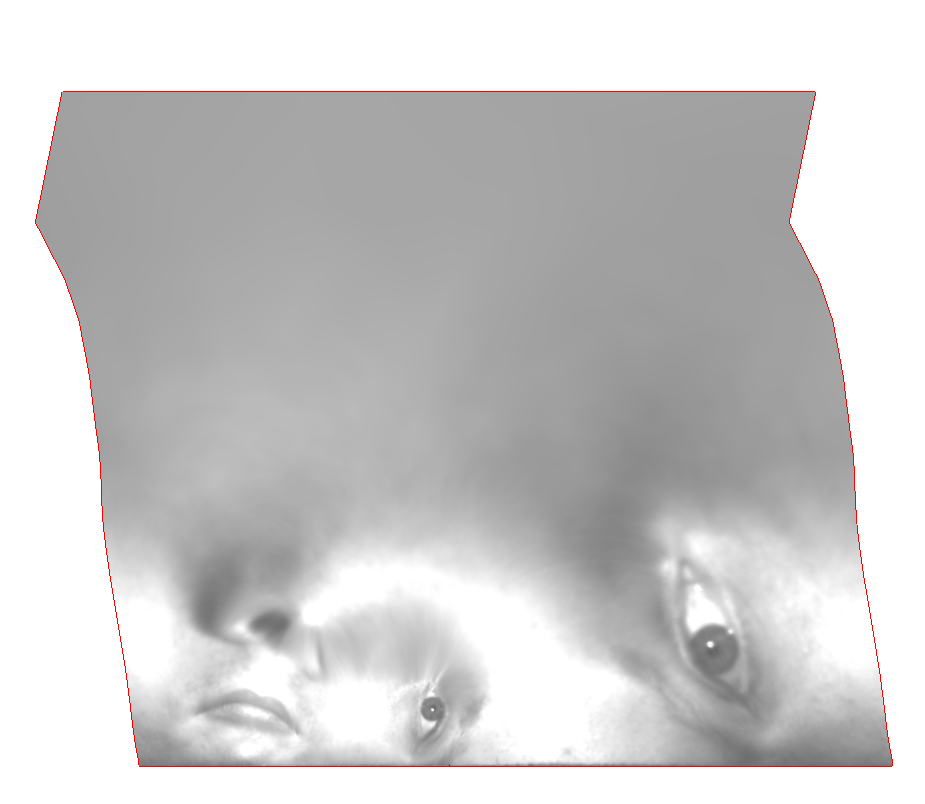The mapping $\phi$.
11. Integration Define a function $\phi: \bar{M}\to C$, choose a base vertex $v_0$, $\phi(v_i) = \int_{v_0}^{v_i} \omega_1 + \sqrt{-1} {}^*\omega_1,$
12.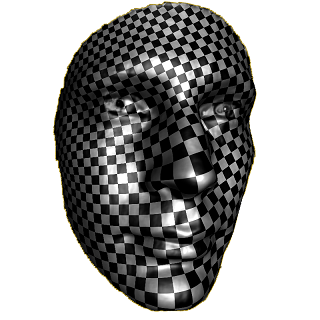Exponential mapping.
13. Exponential MapThe final Riemann mapping is the exponential map of $\phi$, $e^{2\pi \sqrt{-1} \phi(v_i)}$
14. Fill the central hole The central puncture is filled.
15. Mobius Transformation The mapping can be further transformed by a Mobius transformation, $z \to e^{\sqrt{-1}\theta} \frac{z-z_0}{1-\bar{z}_0 z}, |z_0 | < 1.$

### Source Code

The code is written in C++ using VisualStudio on Windows platform. The command pipeline is as follows,
..\..\bin\RiemannMapper.exe -puncture %mesh%.origin.m %mesh%.m
..\..\bin\RiemannMapper.exe -cut %mesh%.m %mesh%
..\..\bin\RiemannMapper.exe -slice %mesh%.cut.m %mesh%.open.m
..\..\bin\RiemannMapper.exe -exact_form %mesh%.m %mesh%
..\..\bin\RiemannMapper.exe -cohomology_one_form_domain %mesh%.m %mesh%.open.m %mesh%_0.dv.m %mesh%_0.dv_uv.m
..\..\bin\RiemannMapper.exe -diffuse %mesh%_0.dv.m %mesh%_0.dv_uv.m %mesh%_0.dv.m %mesh%_0.dv_uv.m
..\..\bin\RiemannMapper.exe -holomorphic_form %mesh%_0.du.m %mesh%_0.dv.m
..\..\bin\RiemannMapper.exe -slit_map holomorphic_form_0.m 0 1 holomorphic.m
..\..\bin\RiemannMapper.exe -integration holomorphic.m %mesh%.open.m %mesh%.uv.m
..\..\bin\RiemannMapper.exe -polar_map %mesh%.m %mesh%.uv.m %mesh%.circ_uv.m
..\..\bin\RiemannMapper.exe -fill_hole %mesh%.circ_uv.m %mesh%.final_uv.m

The source code, testing mesh, texture images and testing scripts can be downloaded here. A simple triangle mesh viewer can be found here. The command line is

RiemannMapper.exe -riemann_map input.m output.m

In order to view the output

MeshViewer.exe output.m checker.bmp

### Contact

If you encounter any problem during the compilation or running the code, please contact the author David Gu at $gu@cs.stonybrook.edu$.